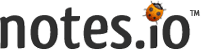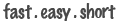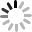# Notes## Notes - notes.io

clc; clear; close all; warning off all;
figure, imshow(Img(:,:,1));
I=Img;
YCbCr = rgb2ycbcr(I);
Y = YCbCr(:,:,1);
Cb = YCbCr(:,:,2);
Cr = YCbCr(:,:,3);
figure, imshow(Y);
I=Y;
d1x = [1 0 -1];
d1y = [1;0-1];
Ix = conv2(I,d1x,'same');
Iy = conv2(I,d1y,'same');
J = sqrt((Ix.^2)+(Iy.^2));
figure, imshow(J,[]);

K = J>26;
L = imclearborder(K);
K=edge(K, 'canny');
figure, imshow(K);
M = imfill(L,'holes');
N = bwareaopen(M,350);
figure, imshow(N);
area = sum(sum(N))

O = bwmorph(N,'remove');
figure, imshow(O);
perimeter = sum(sum(O))

[B,L] = bwboundaries(N,'noholes');
figure,imshow(Img)
hold on
for k = 1:length(B)
boundary = B{k};
plot(boundary(:,2), boundary(:,1), 'y', 'LineWidth', 2)
end
[row,col] = find(N==1);

[a,b] = size(N);

R = Img(:,:,1);
G = Img(:,:,2);
B = Img(:,:,3);

RGB = cat(3,R,G,B);

hsv=rgb2hsv(Img);
v=hsv(:,:,3);

range=[50 600]/750;
se=strel('disk',2);

XY=cat(1,merkez.Centroid);

figure, imshow(RGB),title(['Toplam Balon Sayısı: ',num2str(toplam)]);
crop = imcrop(Img,[min(col) min(row) max(col)-min(col) max(row)-min(row)]);
figure, imshow(crop);Notes.io is a web-based application for taking notes. You can take your notes and share with others people. If you like taking long notes, notes.io is designed for you. To date, over 8,000,000,000 notes created and continuing...

With notes.io;

• * You can take a note from anywhere and any device with internet connection.
• * You can quickly share your contents without website, blog and e-mail.
• * You don't need to create any Account to share a note. As you wish you can use quick, easy and best shortened notes with sms, websites, e-mail, or messaging services (WhatsApp, iMessage, Telegram, Signal).
• * Notes.io has fabulous infrastructure design for a short link and allows you to share the note as an easy and understandable link.

Fast: Notes.io is built for speed and performance. You can take a notes quickly and browse your archive.

Easy: Notes.io doesn’t require installation. Just write and share note!

Short: Notes.io’s url just 8 character. You’ll get shorten link of your note when you want to share. (Ex: notes.io/q )

Free: Notes.io works for 12 years and has been free since the day it was started.

You immediately create your first note and start sharing with the ones you wish. If you want to contact us, you can use the following communication channels;

Email: [email protected]

Instagram: http://instagram.com/notes.io

Regards;
Notes.io Team###### Long File

For written notes was greater than 18KB Unable to shorten.